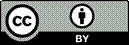Article citation information:

Krasuski, K., Kirschenstein, M. Designation of meteorological parameters using GPS satellite technique in a flight experiment at the Dęblin military aerodrome. Scientific Journal of Silesian University of Technology. Series Transport. 2019, 103, 69-79. ISSN: 0209-3324. DOI: https://doi.org/10.20858/sjsutst.2019.103.6.

Kamil KRASUSKI, Małgorzata KIRSCHENSTEIN

DESIGNATION OF METEOROLOGICAL PARAMETERS USING GPS SATELLITE TECHNIQUE IN A FLIGHT EXPERIMENT AT THE DĘBLIN MILITARY AERODROME

Summary. This paper presents research results on the determination of meteorological parameters utilising the GPS satellite technique. The meteorological parameters were designated using Standard Atmosphere (SA) and UNB3m empirical models. The research experiment was realised during a flight test at the Dęblin military aerodrome. In the flight test, the Cessna 172 plane was used. The values of meteorological parameters (for example, temperature, pressure and relative humidity) from the troposphere empirical models were presented and compared in the paper. In addition, the values of the meteorological parameters were estimated at flight attitude. The range of the flight attitude was between 150 and 700 m. The precision position of the aircraft in vertical frame was determinated using the RTK-OTF differential technique. The mean difference of temperature between the SA and UNB3m models is equal to -5.7°C with the RMS bias approximately 0.2°C. The mean difference of pressure between the SA and UNB3m models equals -1.0 hPa with the RMS bias of approximately 0.3 hPa. The mean difference of relative humidity between the SA and UNB3m models equals 25.5%, with the RMS bias approximately 0.6%. On paper, the values of meteorological data from the SA and UNB3m models were compared with true results interpolated from SYNOP message. In research, the three SYNOP stations, that is, Kozienice, Deblin/Irena and Lublin Radawiec were used for interpolation of the real meteorological data. The difference between empirical and interpolated meteorological data were presented in this paper. The accuracy of the designation of temperature is better in the SA model rather than the UNB3m model. The accuracy of the designation of pressure was relatively low in both models, SA and UNB3m. On the other hand, the accuracy of the designation of relative humidity was better in the UNB3m model than the SA model.

Keywords: GPS, meteorological parameters, SA model, UNB3m model, accuracy

1. INTRODUCTION

The meteorological parameters are applied in the GPS satellite technique for a recovery of such troposphere delay products as Zenith Wet Delay (ZWD) and Zenith Hydrostatic Delay (ZHD). The three major meteorological parameters (that is, temperature, pressure and relative humidity) are necessary for the determination of the troposphere delay . The meteorological parameters can be determined on the basis of data from meteorological stations or equipment. If the measurement data from the meteorological sensors are not available, then meteorological parameters are estimated using empirical models of troposphere. The empirical models include the average value and seasonal variation of meteorological parameters from previous years . The empirical models such as Standard Atmosphere (SA), UNB3m, MOPS, GPT or GPT-2 are implemented in the GPS satellite technique . These empirical models are extremely important in the safety aspect of air navigation. The meteorological parameters from the empirical models can be updated if a GPS receiver is installed in the cockpit. Moreover, the meteorological parameters are corrected based on the aircraft coordinates from an onboard GPS receiver. The corrected meteorological parameters are useful for pilots in order to assess changes in the atmosphere.

In this paper, the meteorological parameters (that is, temperature, pressure and relative humidity) are estimated using the SA and UNB3m empirical troposphere models. The meteorological parameters are designated during the flight test of a Cessna 172 aircraft at Dęblin aerodrome. During the test, the aircraft position was recovered based on GPS observations from a Topcon HiperPro receiver. In the paper, the research results from the SA and UNB3m models were also compared with a true value of the meteorological data of SYNOP data.

2. METHODOLOGY

The meteorological parameters were developed using the SA and UNB3m empirical models. The meteorological parameters in the SA model are expounded thus [2, 4]: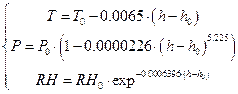(1)

where: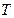- temperature at required height.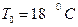.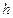- required height.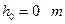.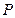- pressure at required height.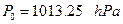.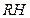- relative humidity at required height.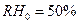.

The meteorological parameters in the UNB3m model are estimated using the mathematical formulas as follows [5, 8]: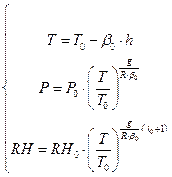(2)

where: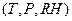- temperature, pressure and relative humidity at required height.- required height.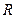- gas constant for dry air.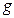- standard acceleration of gravity.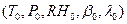- interpolated meteorological parameters.- interpolated temperature, the temperatureis interpolated based on day of year, receiver latitude, average and seasonal variation of temperature from previous years.- interpolated pressure, the pressureis interpolated based on day of year, receiver latitude, average and seasonal variation of pressure from previous years.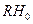- interpolated relative humidity, the relative humidityis interpolated based on day of year, receiver latitude, average and seasonal variation of relative humidity from previous years.- interpolated temperature lapse rate, the temperature lapse rateis interpolated based on day of year, receiver latitude, average and seasonal variation of temperature lapse rate from previous years.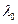- interpolated water vapour lapse rate, the water vapour lapse rateis interpolated based on day of year, receiver latitude, average and seasonal variation of water vapour lapse rate from previous years.

3. RESEARCH EXPERIMENT AND RESULTS

In the research experiment, the meteorological parameters (that is, temperature, pressure and relative humidity) were estimated using the SA and UNB3m models. The meteorological parameters were calculated based on the navigation coordinates of the Cessna 172 aircraft. The aircraft position in the geodetic BLh frame (B- Latitude, L- Longitude and h- ellipsoidal height) was recovered based on GPS observations from a Topcon HiperPro receiver . The aircraft coordinates were determined for the RTK-OTF double differential positioning technique for code and phase measurements. The solving of the aircraft position was conducted in the RTKLIB software in RTKPOST library for “Moving Baseline” module . The final coordinates of the aircraft position in the geodetic frame (that is, ETRF’89) are presented in Figure 1. The flight mission was realised between 09:39:03 and 10:35:03 of the GPS time.

Figure 2 presents values of temperature based on the SA and UNB3m empirical models. The average value of temperature reached 15.1°C in the SA model, and 9.6°C in the UNB3m model, respectively. The minimum value of temperature is close to 13.4°C in the SA model and 8.1°C in the UNB3m model, whereas the ellipsoidal height of the aircraft reaches 700 m. The maximum value of the temperature equals 17.1°C in the SA model and 11.2°C in the UNB3m model, whereas the ellipsoidal height of the aircraft falls down to 148 m. The median value of temperature is approximately 14.2°C in the SA model and 8.8°C in the UNB3m model, respectively.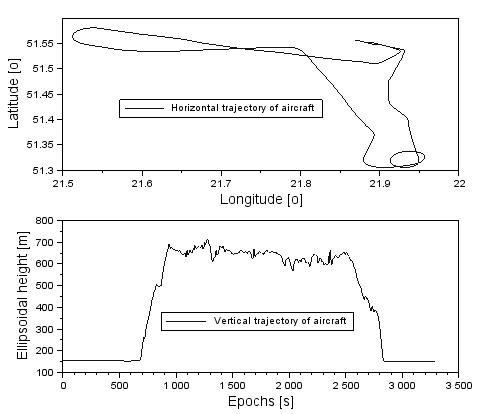Fig. 1. The coordinates of the aircraft in geodetic frame BLh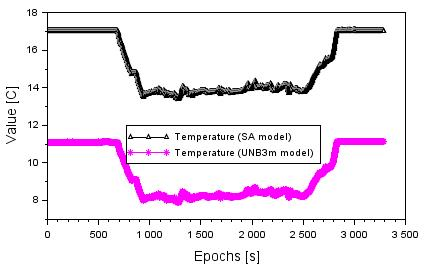Fig. 2. Values of temperature based on SA and UNB3m models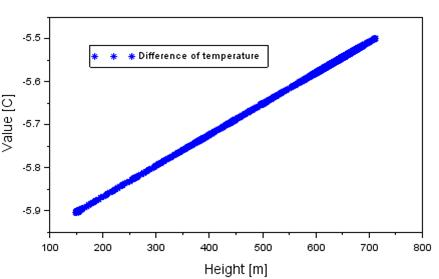Fig. 3. Difference of temperature between SA and UNB3m models

The difference in temperature between the UNB3m and SA models is presented in Figure 3. The mean difference of temperature equals -5.7°C with the RMS bias of approximately 0.2°C. The range of the temperature difference, based on the values from Figure 3, ranges between -5.9 and 5.5°C, respectively. The value of -5.9°C is determined when the height of flight equals 150 m. In addition, the value of -5.5°C is determined when the height of flight equals 710 m.

Figure 4 presents the values of pressure based on the SA and UNB3m empirical models. The average value of pressure equals 961.8 hPa in the SA model, and 960.9 hPa in the UNB3m model, respectively. The minimum value of pressure is close to 940.0 hPa in the SA model and 929.5 hPa in the UNB3m model, whereas the ellipsoidal height of aircraft grows up to 700 m. The maximum value of pressure equals 995.6 hPa in the SA model and 994.9 hPa in the UNB3m model, whereas the ellipsoidal height of the aircraft falls down to 148 m. The median value of pressure is approximately 944.4 hPa in the SA model and 943.2 hPa in the UNB3m model, respectively.

The difference of pressure between the UNB3m and SA models is presented in Figure 5. The mean difference of pressure equals -1.0 hPa with the RMS bias of approximately 0.3 hPa. The range difference is between -1.4 and -0.6 hPa, respectively. The value of -0.6 hPa is determined when the height of flight equals 150 m. In addition, the value of -1.4 hPa is determined at an altitude of 710 m.Fig. 4. Values of pressure based on SA and UNB3m models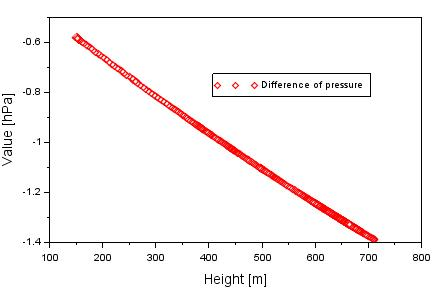Fig. 5. Difference of pressure between SA and UNB3m models

Figure 6 presents values of relative humidity parameter based on the SA and UNB3m models. The average value of relative humidity term from the SA model equals 38.1%, with a range between 31.7 and 45.5%. The average value of the relative humidity term from the UNB3m model equals 63.1%, with a range between 55.9 and 71.1%. The median value of relative humidity parameter reaches 34.2% from the SA model and 58.5% from the UNB3m model, respectively.

The differences in relative humidity between the results from the SA and UNB3m models are presented in Figure 7. The average value of difference of relative humidity is approximately -25.5%, with magnitude order between -25.9 and -24.9%, respectively. The RMS bias of difference of relative humidity term equals 0.6%. In addition, the median value of difference of relative humidity equals approximately -25.4%. The value of -25.9 hPa is determined when the height of flight equals 150 m. In addition, the value of -24.9 hPa is determined when the altitude equals 710 m.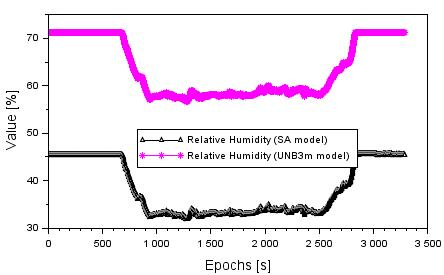Fig. 6. Values of relative humidity based on the SA and UNB3m models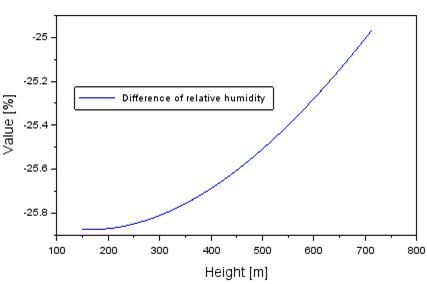Fig. 7. Difference of relative humidity between the SA and UNB3m models

4. DISCUSSION

A comparative presentation of the research results with model readings of meteorological parameters based on data from the SYNOP message is given. In the research, the authors used the actual measurement data of the SYNOP stations, belonging to the IMGW in Warsaw. In particular, the authors used meteorological data from the following stations:

*) SYNOPS from 12488, Kozienice (Poland) | 51-34N | 021-33E | 107 m.

**) SYNOPS from 12490, Deblin / Irena (Poland) | 51-33N | 021-51E | 124 m.

***) SYNOPS from 12495, Lublin Radawiec (Poland) | 51-13N | 022-24E | 238 m.

The metrological data SYNOP for the station Kozienice, Deblin/Irena and Lublin Radawiec were downloaded from the web service, www.ogimet.com . The meteorological stations Kozienice, Deblin/Irena and Lublin Radawiec were the closest to the flight trajectory of the aircraft, which resulted in their selection in the flight test. In the first order, the distribution of meteorological parameters at the SYNOP stations was specified for the interval of the observation time during the duration of the flight test. Thus, it was possible to determine the values of the meteorological parameters over the stations of Kozienice, Deblin/Irena and Lublin Radawiec, in accordance with the data contained in the message SYNOP. Thereafter, an interpolation of meteorological parameters was made from the level of reference elevation of Kozienice, Deblin/Irena and Lublin Radawiec stations to the Cessna 172 flight altitude. In the calculations, the authors used the interpolation of meteorological data, in accordance with the mathematical formulas discussed in this study .

Within the discussions, it was possible to determine the accuracy of the exploited models, SA and UNB3m, in the designation of metrological parameters. The accuracy of the models, SA and UNB3m, was found in relation to the metrological parameters, determined by means of the interpolation method based on the data from the message SYNOP. In this way, the difference in metrological parameters was designated, as below: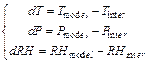(3)

where: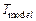- temperature from the SA and UNB3m model, based on equations (1) and (2).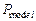- pressure from the SA and UNB3m model, based on equations (1) and (2).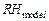- relative humidity from the SA and UNB3m models, based on equations (1) and (2).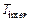- temperature interpolated from SYNOP data.- pressure interpolated from the SYNOP data.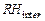- relative humidity interpolated from the SYNOP data.

Figure 8 shows the accuracy of determining temperature based on the SA and UNB3m models in relation to the interpolated readings from the SYNOP station. The temperature accuracy from the UNB3m model ranges from18.6 to -4.1°C. In addition, the average accuracy value of the temperature from the model UNB3m equals -5.7°C, whereas the RMS error is equal to 1.1°C. The temperature accuracy from the SA model ranged from -1.6 to +1.9°C. In addition, the average accuracy value of the temperature of the SA model is +0.1°C, whereas the RMS error is equal to 1.3°C.

Figure 9 shows the accuracy of determining pressure from the SA and UNB3m models, in relation to the interpolated readings from the SYNOP station. The accuracy of temperature from the model UNB3m ranges from -61.3 to +4.3 hPa. In addition, the mean value of the temperature accuracy from the UNB3m model equals -29.3 hPa, whereas the RMS error is equal to 26.6 hPa. The accuracy of pressure from the SA model ranges from -59.9 to +4.8 hPa. Moreover, the mean value of temperature accuracy from the SA model equals -28.3 hPa, whereas the RMS error is equal to 25.9 hPa.

Figure 10 shows the accuracy of determining relative humidity from the SA and UNB3m models, in relation to the interpolated readings from the SYNOP station. The accuracy of relative humidity of the UNB3m model is between -28.6 and -9.1%. In addition, the mean value of the relative humidity value from the UNB3m equals -20.3%, with the RMS error being equal to 8.1%. The accuracy of relative humidity of the SA model is between -53.5 and -35.1%. Furthermore, the mean value of the relative humidity value from the SA equals -45.9%, whereas the RMS error is equal to 7.8%.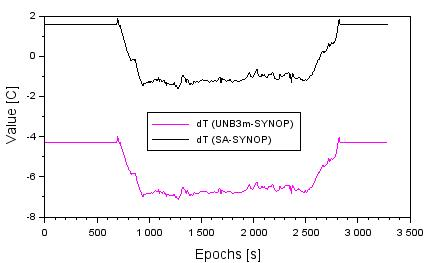Fig. 8. The accuracy of temperature from SA and UNB3m models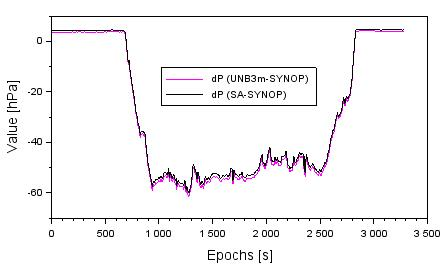Fig. 9. The accuracy of pressure from SA and UNB3m models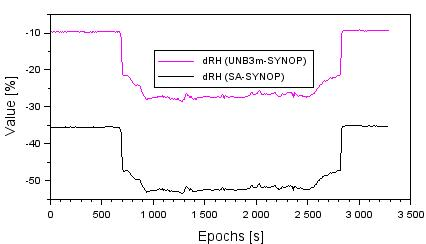Fig. 10. The accuracy of relative humidity from SA and UNB3m models

5. CONCLUSIONS

The article presents practical applications of the empirical troposphere models in order to determine the meteorological parameters, that is, temperature, pressure and relative humidity. The article uses two troposphere models: the SA model and the UNB3m model. The article presents two algorithms of meteorological parameters, in the SA and UNB3m models. Investigations were conducted within the test flight performed by the aircraft Cessna 172 for the military aerodrome EPDE in Dęblin. The designated meteorological parameters for the SA and UNB3m models were determined for the Cessna 172 flight trajectory. In addition, this paper determines the accuracy of determined meteorological parameters in relation to the actual readings from the SYNOP message. The actual values of the meteorological parameters were designated by means of interpolation, on the basis of data from the SYNOP stations of Kozienice, Deblin/Irena and Lublin Radawiec. The accuracy of the designation of temperature is better in the SA model than the UNB3m model. The accuracy of the designation of pressure is relatively low in both the SA and UNB3m models. On the other hand, the accuracy of the designation of relative humidity is better in the UNB3m model than the SA model. It is worth adding that the models of the meteorological parameters from the SA and UNB3m models are used in GPS satellite measurements and exploited in aviation.

Acknowledgement

The authors would like to thank the OGIMET service for making its meteorological data available.

References

1.             Bosy J., A. Borkowski. 2005. Troposphere modeling in local GP network. Paper presented at: EUREF Symposium 2005 Vienna, length of paper: 11.

2.             Bosy J. 2005. Precise processing of satellite GPS observations in local networks located in mountain areas. Publisher of the Agricultural University in Wroclaw. No 522. ISSN 0867–7964.

3.             Ciećko A., G. Grunwald, R. Kaźmierczak, M. Grzegorzewski, J. Ćwiklak, S. Oszczak, M. Bakuła. 2014. „Analysis of the accuracy and availability of ASG-EUPOS services in air navigation and transport”. Logistyka 3: 1091-1100.

4.             Dach R., U. Hugentobler, P. Fridez, M. Meindl. 2007. Bernese GPS software version 5.0. Astronomical Institute, University of Bern.

5.             Isioye O.A., L. Combrinck, J. Botai. 2015. “Performance evaluation of Blind Tropospheric delay correction models over Africa”. South African Journal of Geomatics 4(4): 502-525.

6.             Kowalski M. 2012. “Phase mapping in the diagnosing of a turbojet engine”. Journal of theoretical and applied mechanics 50(4): 913-921.

7.             Kozakiewicz A., M. Kowalski. 2013. “Unstable operation of the turbine aircraft engine”. Journal of theoretical and applied mechanics 51(3): 719-727.

8.             Leandro R.F., R.B. Langley, M.C. Santos. 2008. “UNB3m_pack: a neutral atmosphere delay package for radiometric space techniques”. GPS Solutions 12: 65-70. DOI: 10.1007/s10291-007-0077-5.

9.             Sanz Subirana J., Juan Zornoza J.M., Hernández-Pajares M. 2013. GNSS Data Processing, Volume I: Fundamentals and Algorithms. Publisher: ESA Communications. ESTEC, Noordwijk, Netherlands. ISBN 978-92-9221-886-7, 2013.

10.         Takasu T. 2013. RTKLIB ver. 2.4.2 Manual, RTKLIB: An Open Source Program Package for GNSS Positioning. Available at: http://www.rtklib.com/prog/manual_2.4.2.pdf.

11.         OGIMET. Available at: http://www.ogimet.com.

12.         Zieja Mariusz, Paweł Gołda, Mariusz Żokowski, Paweł Majewski. 2017. „Vibroacoustic technique for the fault diagnosis in a gear transmission of a military helicopter”. Journal of Vibroengineering 19(2): 1039-1049.

Received 13.01.2019; accepted in revised form 02.05.2019